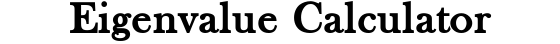##### Related Content

Programmers and Teachers:Nikkolas K.
Tutor and degreed Aerospace Engineer

# Eigenvalue CalculatorSize:
A =

## Eigenvalues Lesson

### What is an Eigenvalue?

The eigenvalues of a matrix are a set of scalars, whereas the eigenvectors of a matrix are a set of vectors. Finding the eigenvalues of a system of equations/matrix is used often in physics and engineering problems. A couple examples of this are solid rotating bodies and vibrating systems. The eigenvalues and eigenvectors help describe these complex mechanical systems that we study in mathematics, physics and engineering.

“Eigen” is German for “own” which is why each eigenvalue has a corresponding eigenvector, and vice versa. The eigenvalues and eigenvectors of any linear system of equations/matrix can be found if matrix is square. A square matrix is one that has an equal number of rows and columns. Non-square matrices only have non-real eigenvalues (they will be imaginary or complex).

It is common for there to be a greater number of eigenvectors than eigenvalues for a given system of equations/matrix. When this happens, there will be multiple eigenvectors per eigenvalue. Therefore, we can view the eigenvalues as the “parents” and the eigenvectors as the “children” since each parent may have one or more children associated with it.

### How to Hand Calculate Eigenvalues

The basic equation representation of the relationship between an eigenvalue and its eigenvector is given as Av = λv where A is a matrix of m rows and m columns, λ is a scalar, and v is a vector of m columns. In this relation, true values of v are the eigenvectors, and true values of λ are the eigenvalues. For something to be a true value, it must satisfy the equation.

The previous equation Av = λv can be rearranged to AI = 0 where I is the identity matrix. Then, we can proceed to carrying out the matrix multiplication and subtraction operations which will result in a polynomial. This polynomial is set equal to zero. Then, the roots of the terms can be solved for. The roots of these terms are the eigenvalues.

In a matrix of m columns and rows, there can be as few as zero eigenvalues, and as many as m eigenvalues. The eigenvalues can be real or complex. Complex eigenvalues will have a real component and an imaginary component.

If we want to also find the associated eigenvectors, we use the original equation Av = λv and plug in the value of each eigenvalue. Then, we solve for every possible value of v. The values we find for v are the eigenvectors.

## How the Calculator Works

This calculator is written in JavaScript (JS), a programming language that has the ability to run inside your device’s internet browser. Because it runs inside the browser, calculations happen immediately when you click “calculate”. There is no waiting on communications to and from a remote server or for the page the reload with data from the server.

The calculator’s core is powered by a numerical routine called the Jacobi method. The Jacobi method iterates through very many approximations until it converges on an accurate solution. In general, numerical routines solve systems of equations/matrices by performing an approximated calculation very many times. The alternative to numerical computation is called symbolic computation. Symbolic routines preserve exact values and use a combination of analytical formulas and steps to solve for an exact solution.

Because computer processors are so powerful at basic math compared to humans, symbolic routines are not needed for solving eigenvalues. The Jacobi method can go through its numerical routines within a fraction of a second and return eigenvalues that are accurate to a minimum of the fifth decimal place. In reality, the computations preserve many more than five decimal places. However, the final answer is chopped down for ease of use and practicality.

This calculator finds the eigenvalues and eigenvectors simultaneously, but only shows the eigenvalues because reporting the eigenvectors can become messy for large matrices. If you would like to also see the eigenvectors of your matrix, visit our eigenvector calculator.

Scroll to Top